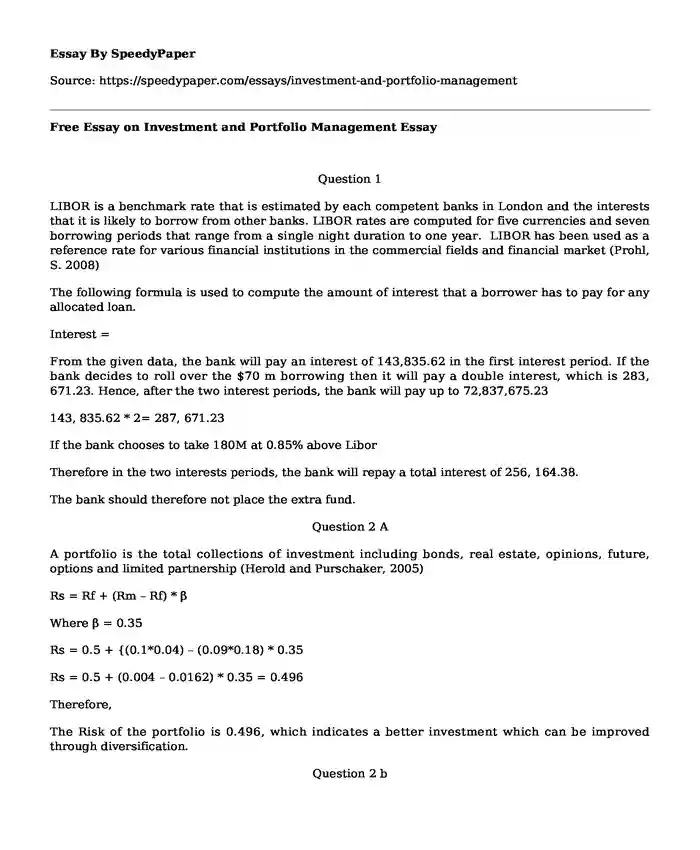# Free Essay on Investment and Portfolio Management

Published: 2017-10-09Type of paper: Problem solving Categories: Management Problem solving Pages: 3 Wordcount: 575 words
143 views

Question 1

Is your time best spent reading someone else’s essay? Get a 100% original essay FROM A CERTIFIED WRITER!

LIBOR is a benchmark rate that is estimated by each competent banks in London and the interests that it is likely to borrow from other banks. LIBOR rates are computed for five currencies and seven borrowing periods that range from a single night duration to one year. LIBOR has been used as a reference rate for various financial institutions in the commercial fields and financial market (Prohl, S. 2008)

The following formula is used to compute the amount of interest that a borrower has to pay for any allocated loan.

Interest =

From the given data, the bank will pay an interest of 143,835.62 in the first interest period. If the bank decides to roll over the \$70 m borrowing then it will pay a double interest, which is 283, 671.23. Hence, after the two interest periods, the bank will pay up to 72,837,675.23

143, 835.62 * 2= 287, 671.23

If the bank chooses to take 180M at 0.85% above Libor

Therefore in the two interests periods, the bank will repay a total interest of 256, 164.38.

The bank should therefore not place the extra fund.

Question 2 A

A portfolio is the total collections of investment including bonds, real estate, opinions, future, options and limited partnership (Herold and Purschaker, 2005)

Rs = Rf + (Rm – Rf) * β

Where β = 0.35

Rs = 0.5 + {(0.1*0.04) – (0.09*0.18) * 0.35

Rs = 0.5 + (0.004 – 0.0162) * 0.35 = 0.496

Therefore,

The Risk of the portfolio is 0.496, which indicates a better investment which can be improved through diversification.

Question 2 b

Portfolio returns = E(R) = w1R1 + w2Rq + ...+ wnRn

E (R) = 0.33 * 0.18 + 0.33* 0.34 + 0.33 * x = 0.05

= 0.0594 + 0.1122 +0.33x = 0.05

x = - = 0.15 – 0.52 = - 0.37

New Risk of the portfolio

+ = 0.07

0.18-0.07= 0.11

0.34- 0.07= 0.27

This indicates that Simon’s company has a previous investment has depicted a lackluster return. However, the new Risk portfolio works much better than the previous investment. There is a better step towards improvement of the investment.

Question 2 C

Expected Return

ERP = Weighted Average of Returns

= R1W1 + R2W2

For bonds

Rs = Rf + (Rm – Rf) * β

β =

Rs = 0.2 + (0.27- 0.2) * 0.18 = 0.434

For Shares

Rs = Rf + (Rm – Rf) * β

Rs = 0.2 + (0.27 – 0.2) * 0.34 = 0.402

ERP = Rf + (Rm – Rf) * βp

βp = β1 w1 + β2 w2

βp = 0.18 * 0.5 + 0.34 * 0.5

βp = 0.09 + 0.17 = 0.26

ERP = 0.2 + (0.9 – 0.2) * 0.26

ERP = 1.048

The positive β Value of 0.26 means that the securities are undervalued.

This also means that 1 % change in the market portfolio causes a 1.048 % change in Simon’s portfolio.

References

Herold, U., Maurer, R. and Purschaker, N. (2005) Total Return Fixed-Income Portfolio Management.The Journal of Portfolio Management, 31(3), pp.32-43

Prohl, S. (2008). Libor Market Models. SSRN Electronic Journal.

Free Essay on Investment and Portfolio Management. (2017, Oct 09). Retrieved from https://speedypaper.com/essays/investment-and-portfolio-management

Request Removal

If you are the original author of this essay and no longer wish to have it published on the SpeedyPaper website, please click below to request its removal:

Liked this essay sample but need an original one?

Hire a professional with VAST experience!